# Week 4: Convolutional Neural Networks

Abstract:

This lecture will introduce convolutional neural networks.

from conv_imports import *
%matplotlib notebook

## Plan for the Day

• Introduction
• Inductive bias
• Inductive bias in NNs
• Convolutional operator
• Translation invariance in images
• Convolutional Neural Networks
• Parameter-free convolution
• Depth-wise separability & shuffle
• Deconvolution & super resolution
• Graph CNNs

## Inductive bias

What is inductive bias?

• An assumption that allows the model to make predictions about never-seen data.
• All models carry one form of an inductive bias or other.
• i.e.: linear model, nonlinear model, type of non-linearity...

Why do we need it?

• Data is expensive to gather or generate.
• Data is sparse - usually, we observe only a sample of the true data population.
• Generalization is necessary for both academic and industrial use of (deep) models.

## Inductive bias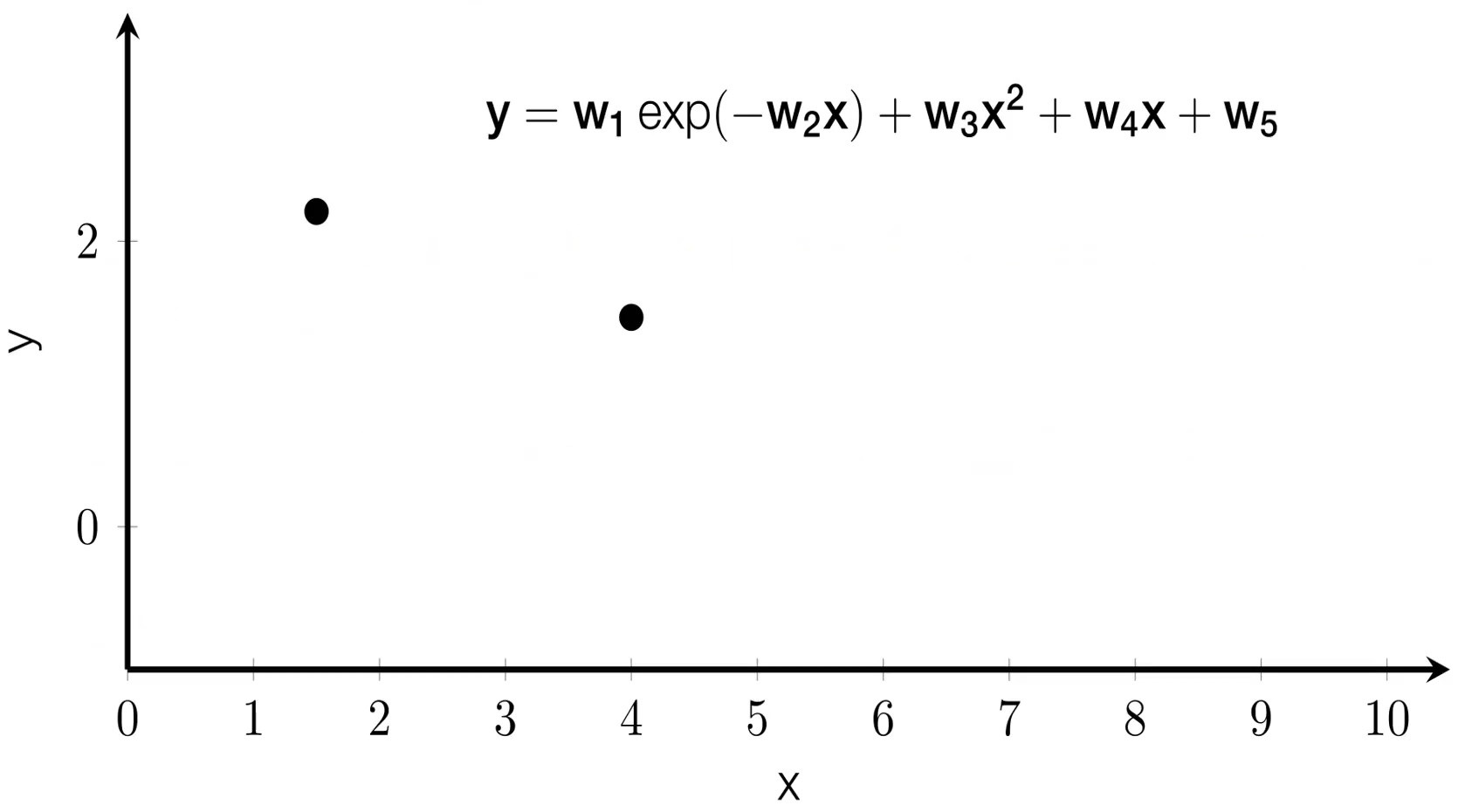## Inductive bias in NNs

A simple universal approximator that makes no assumptions about its input structure.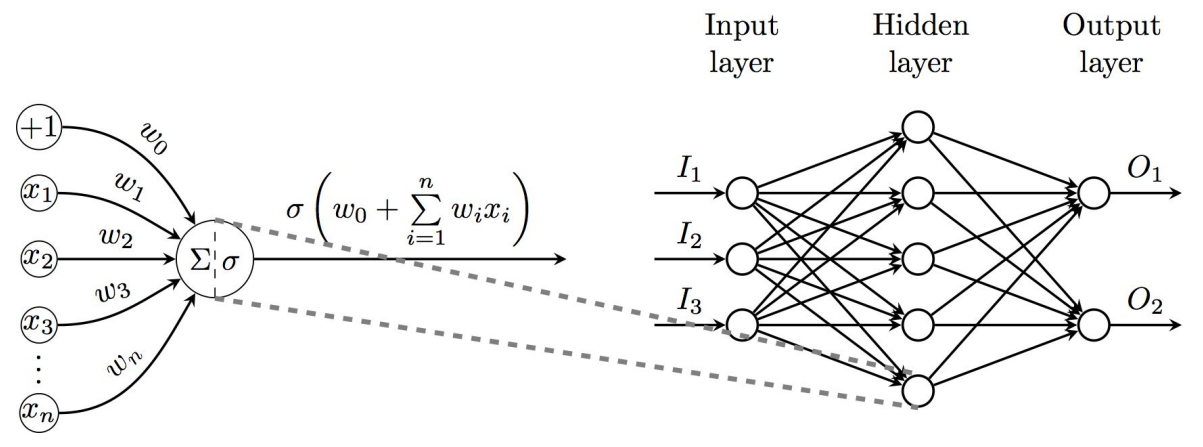## Inductive bias in NNs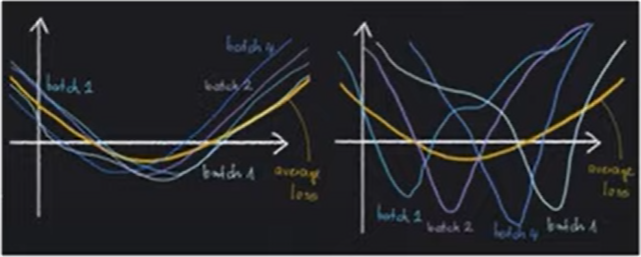• Common to all NNs
• Layered feature maps
• Nodes within a layer are assumed never to interact
• Inputs are processed on an directed acyclic graph
• Preference for models trainable by gradient
• descent given the chosen initializer
• Recall week 2: Optimization and Stochastic Gradient Descent
• Layer-specific inductive biases - relational inductive biases
• Locality: CNNs - preferred model is invariant to spatial translations
• Sequentiality: RNNs - preferred model is invariant to time translations
• Arbitrary: Graph NNs - preferred model is invariant to node & edge permutations

## Inductive bias in NNs

• Plain English: An inductive bias allows a learning algorithm to prioritize one solution (or interpretation) over another, independent of the observed data.

• Bayesian perspective: inductive biases are captured by the model’s prior distribution. In this context, the specific shoice of an NN architecture can be seen as an extreme choice of a prior.

$\large p(\theta\|X,Y)= \huge \frac{p(Y|X,\theta) p(\theta)}{p(Y|X)}$

• Technical perspective: all NN layers can be seen as special cases of fully connected layers. Inductive biases are expressed by the weight-sharing restrictions that define any given layer type.

## Plan for the day

• Introduction
• Inductive bias
• Inductive bias in NNs
• Convolutional operator
• Translation invariance in images
• Convolutional Neural Networks
• Parameter-free convolution
• Depth-wise separability & shuffle
• Deconvolution & super resolution
• Graph CNNs

## Translation invariance in CNNs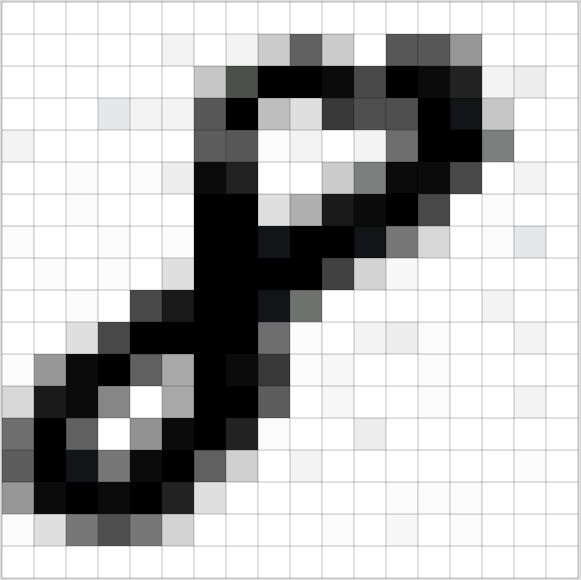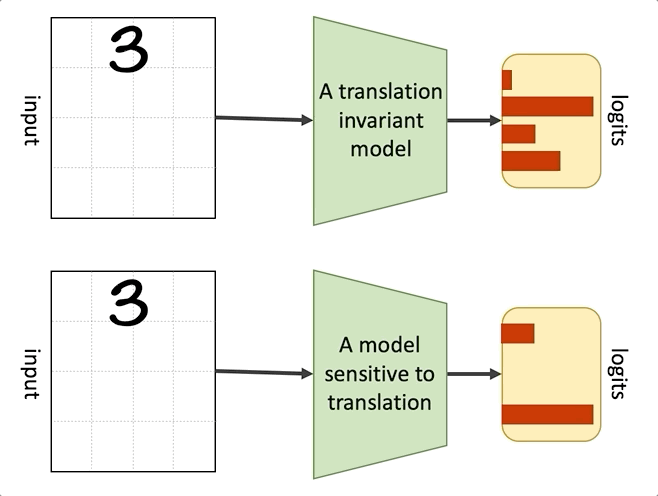## Convolutional Neural Networks

$\large Out_{i,j}= b + \sum_{x=1}^3 \sum_{y=1}^3 In_{(i+x-1),(j+y-1)}W_{x,y}$

Image Kernel Output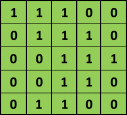## Convolutional Neural Networks

$\large D_{out} = \huge \frac{(D_{in} - K + 2P)}{2} \large + 1$

Kernel size (3x3) Stride (2) Padding (1)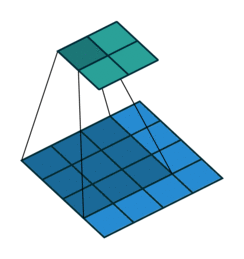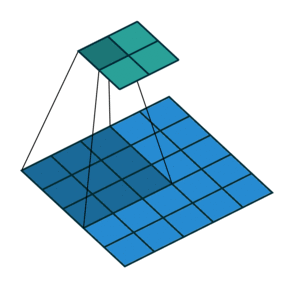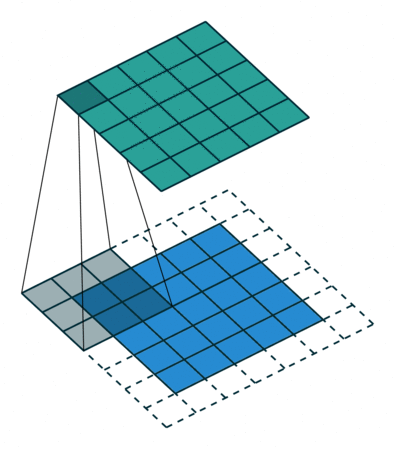## Weight sharing in CNNs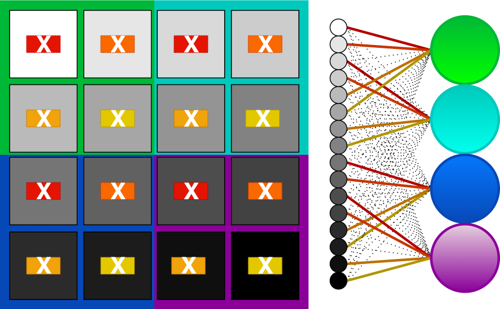## Numerical example of a CNN - code

image = show_car(CIFAR10_trainset)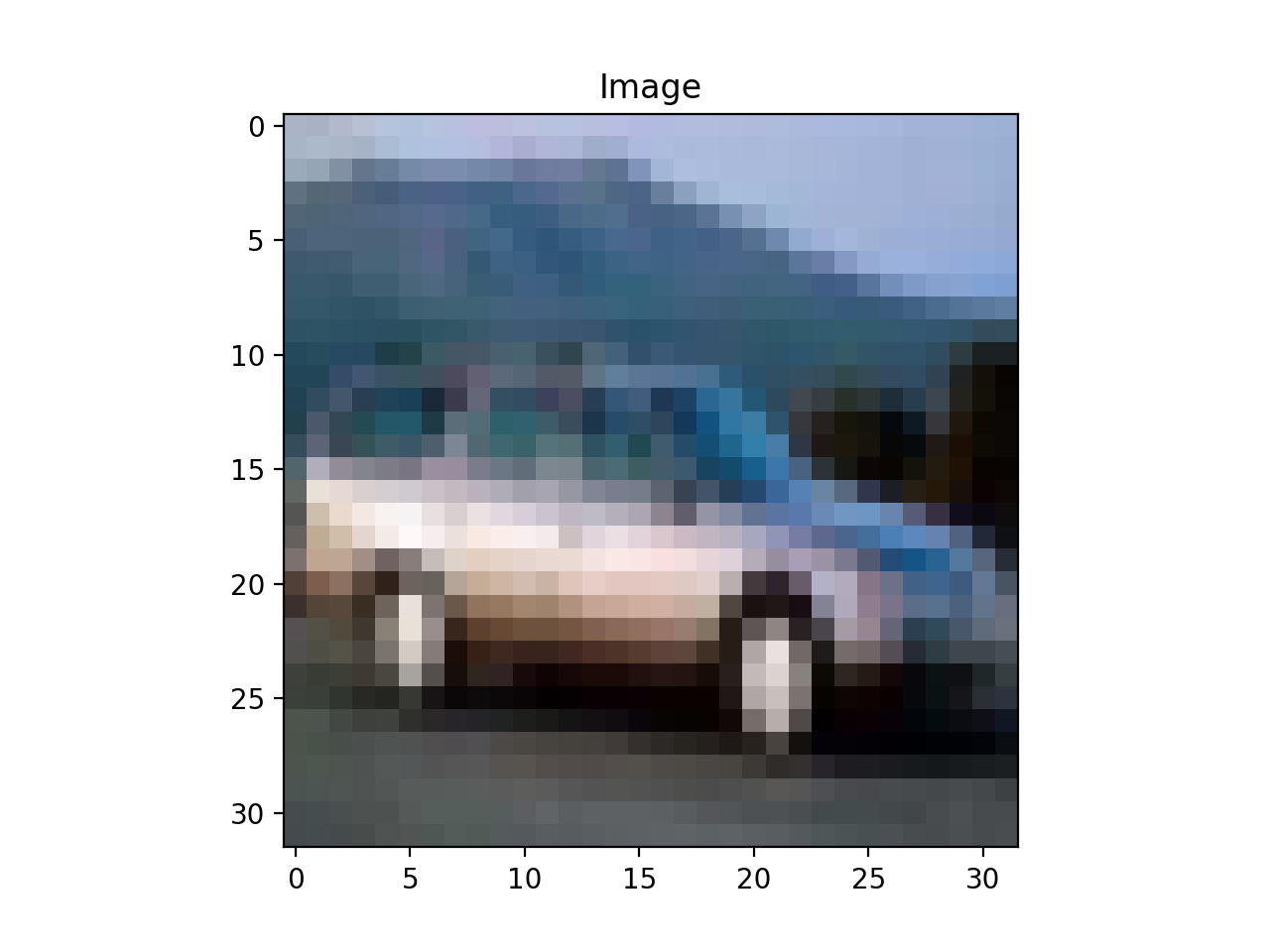## Numerical example of a CNN - code

conv = show_random_kernel()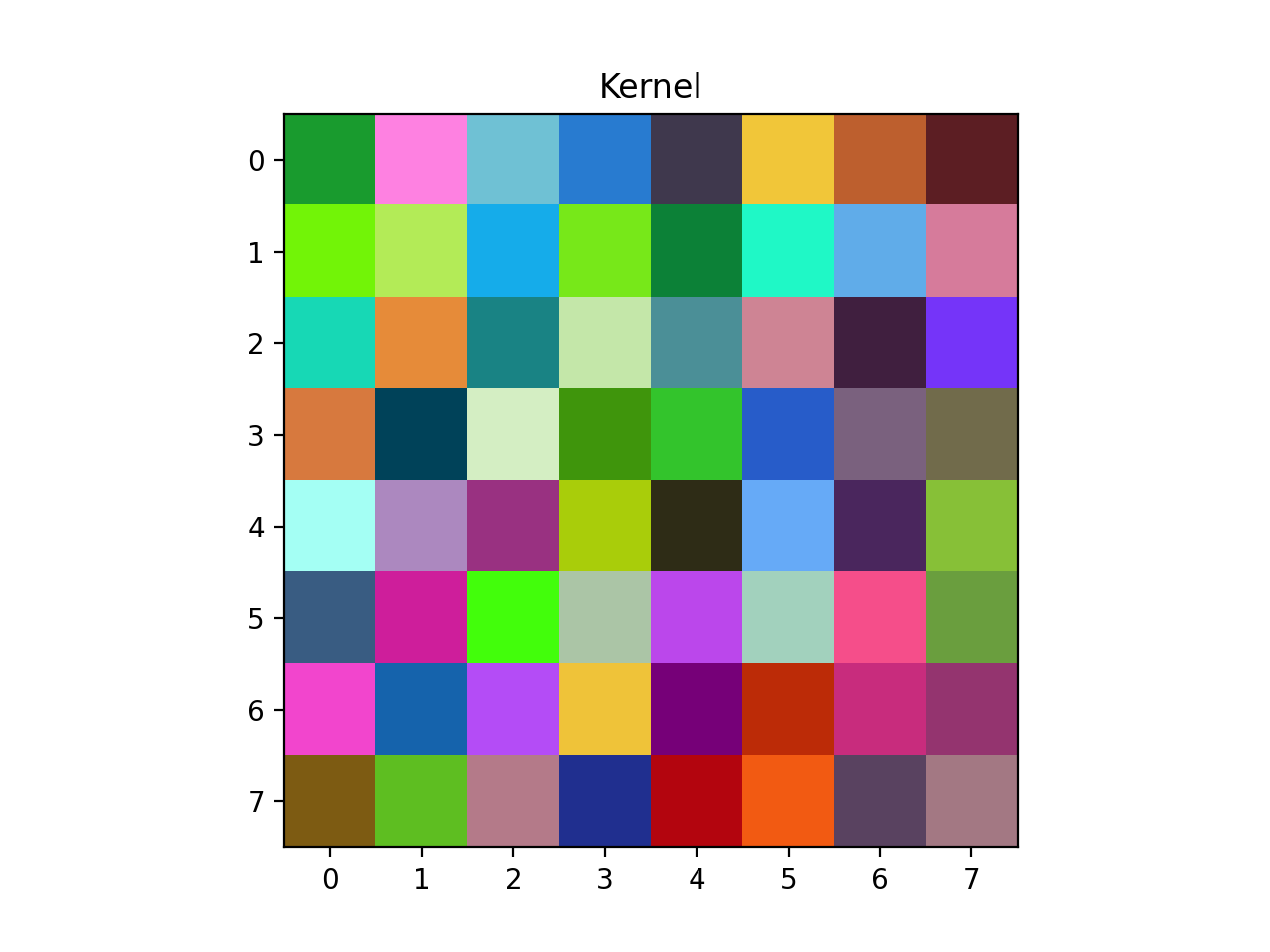## Numerical example of a CNN - code

fig, num_steps, im, final_fm = show_conv_anim(image, conv)
anim = animation.FuncAnimation(fig, update_image, num_steps,
fargs=(fig, im, final_fm), interval=200, blit=True)## CNN as an edge detector

image = show_cute_fox()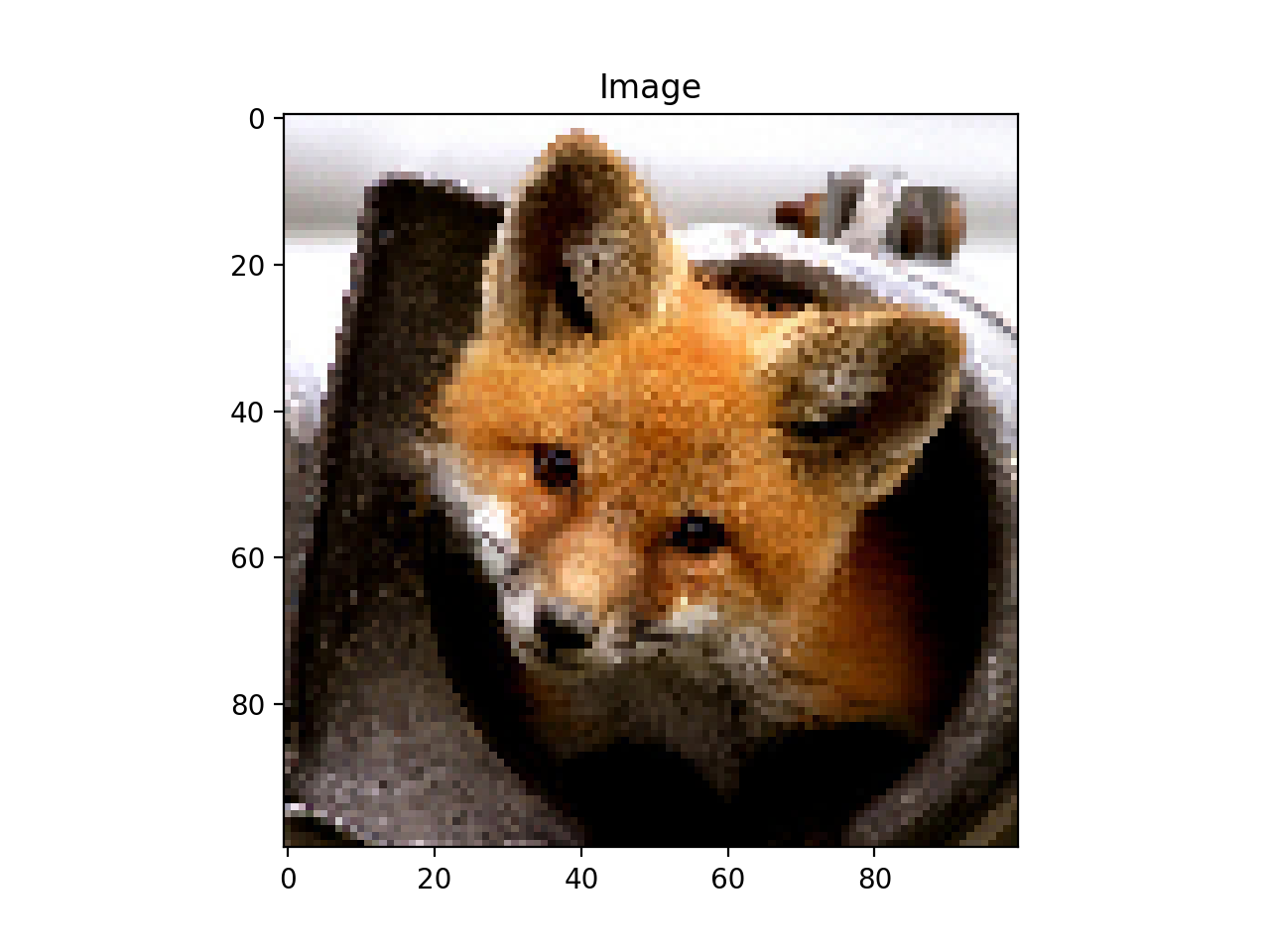## CNN as an edge detector

kernel = np.array([[0,-1,0],
[-1,4,-1],
[0,-1,0]])
conv = show_edge_detector(kernel.T)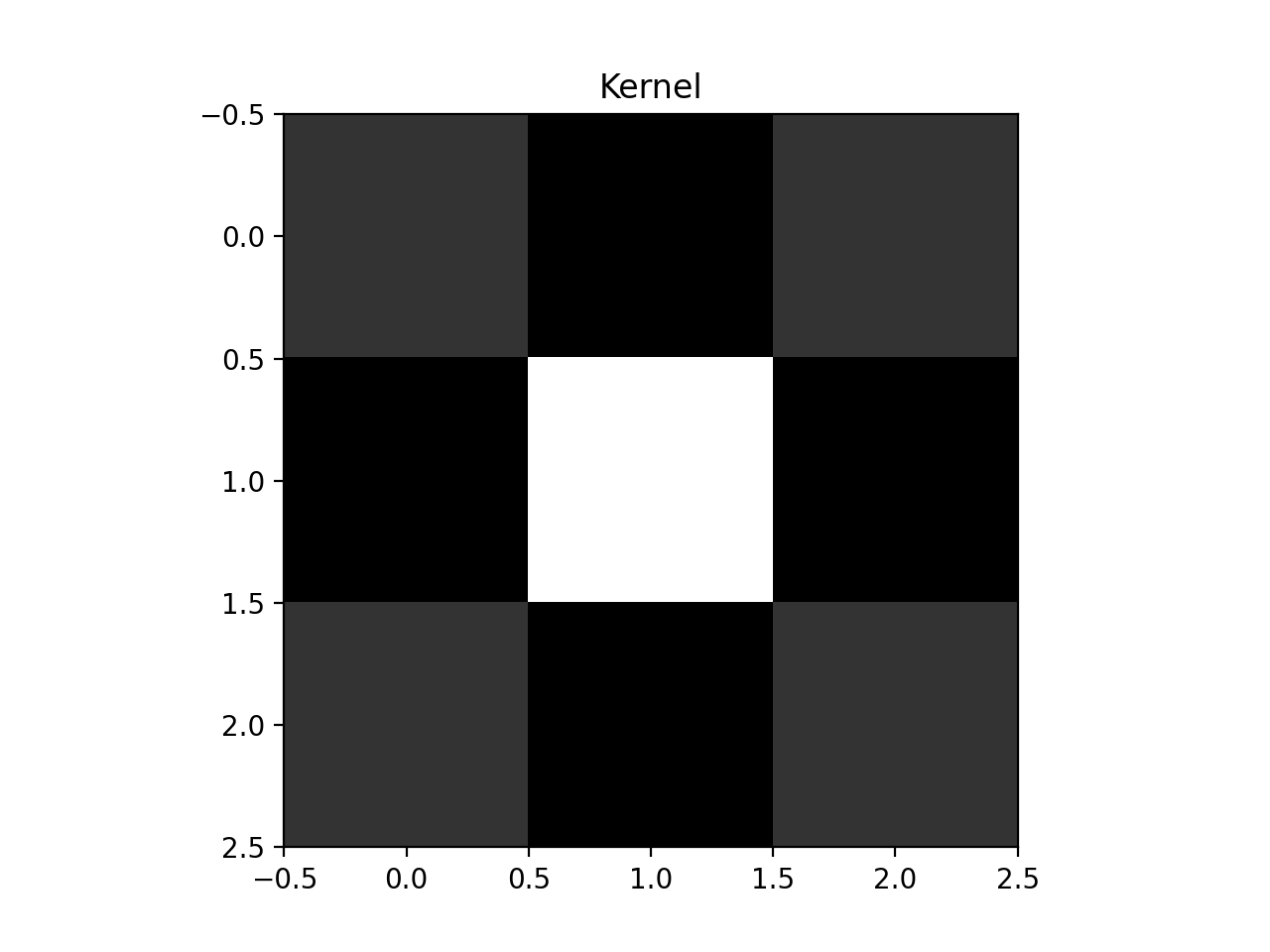## CNN as an edge detector

fig, num_steps, im, final_fm = show_edge_detection(image, conv)
anim = animation.FuncAnimation(fig, update_image_no_annotation, range(0, num_steps, 500), fargs=(im, final_fm),
interval=100, blit=True)## Learned CNN features - setup

convlayer, bnlayer, relulayer = get_mobilenet_convbnrelu()
blue_fox = show_224px_fox()
Downloading: "https://download.pytorch.org/models/mobilenet_v2-b0353104.pth" to /root/.cache/torch/hub/checkpoints/mobilenet_v2-b0353104.pth
{"model_id":"e55d4ca4ad03451db55cbc25eb7d423a","version_major":2,"version_minor":0}
<IPython.core.display.Javascript object>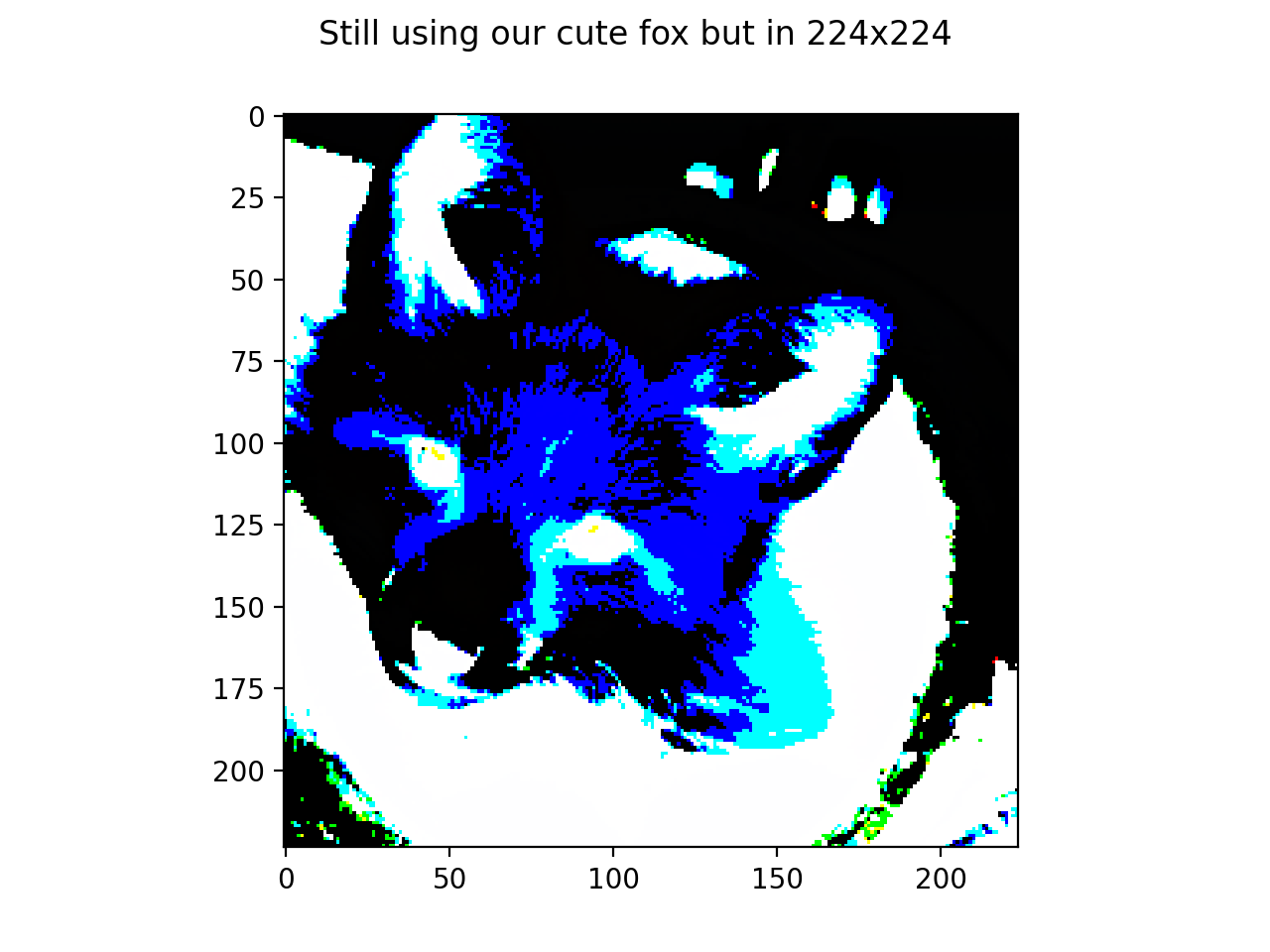## Learned CNN features - weights at layer 3

fig, num_steps, ims = show_conv_weights(convlayer)
anim = animation.FuncAnimation(fig, update_row, num_steps,
fargs=(ims, convlayer.weight), interval=1000, blit=True)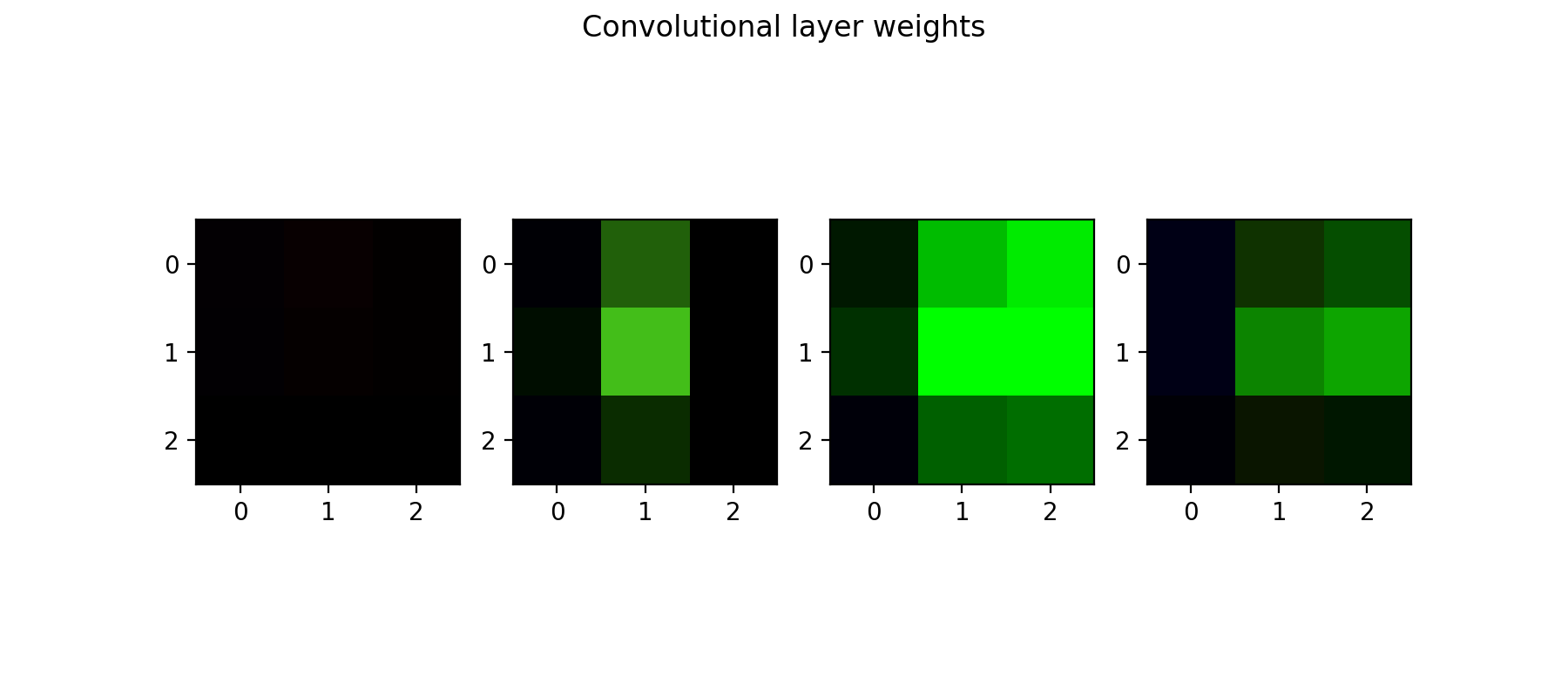## Learned CNN features - convolution pre-activation at layer 3

fig, num_steps, ims, convoutput = show_layer_output(blue_fox, convlayer)

anim = animation.FuncAnimation(fig, update_row_output, num_steps,
fargs=(ims, convoutput), interval=1000, blit=True)